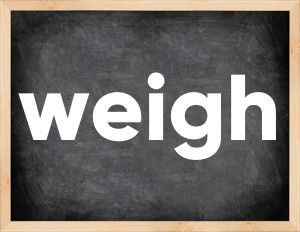# Weigh past tenseThe English verb 'weigh' is pronounced as [weɪ].
Related to: regular verbs.
3 forms of verb weigh: Infinitive (weigh), Past Simple - (weighed), Past Participle - (weighed).

## Here are the past tense forms of the verb weigh

👉 Forms of verb weigh in future and past simple and past participle.
❓ What is the past tense of weigh.

## Weigh: Past, Present, and Participle Forms

Base Form Past Simple Past Participle
weigh [weɪ]

weighed [weɪd]

weighed [weɪd]

## What are the 2nd and 3rd forms of the verb weigh?

🎓 What are the past simple, future simple, present perfect, past perfect, and future perfect forms of the base form (infinitive) 'weigh'?

### Learn the three forms of the English verb 'weigh'

• the first form (V1) is 'weigh' used in present simple and future simple tenses.
• the second form (V2) is 'weighed' used in past simple tense.
• the third form (V3) is 'weighed' used in present perfect and past perfect tenses.

## What are the past tense and past participle of weigh?

The past tense and past participle of weigh are: weigh in past simple is weighed, and past participle is weighed.

### What is the past tense of weigh?

The past tense of the verb "weigh" is "weighed", and the past participle is "weighed".

### Verb Tenses

Past simple — weigh in past simple weighed (V2).
Future simple — weigh in future simple is weigh (will + V1).
Present Perfect — weigh in present perfect tense is weighed (have/has + V3).
Past Perfect — weigh in past perfect tense is weighed (had + V3).

### weigh regular or irregular verb?

👉 Is 'weigh' a regular or irregular verb? The verb 'weigh' is regular verb.

## Examples of Verb weigh in Sentences

•   Last thing I captained weighed 22000 tons. (Past Simple)
•   We've weighed the container (Present Perfect)
•   He carefully weighed all pros and cons before making the decision. (Past Simple)
•   The butcher weighed the cow. (Past Simple)
•   He weighed his son on the scale. (Past Simple)
•   She weighed 4 kilos at birth. (Past Simple)
•   The seller have weighed out half a kilo of rice. (Present Perfect)
•   Why does this point deserves to be carefully weighed? (Present Simple)
•   He weighed the ball in his hand. (Past Simple)
•   She weighed the consequences of marrying him.. (Past Simple)

Along with weigh, words are popular go through with and sign in.

Verbs by letter: , , , , , , , , , , , , , , , , , , , , , , , , .